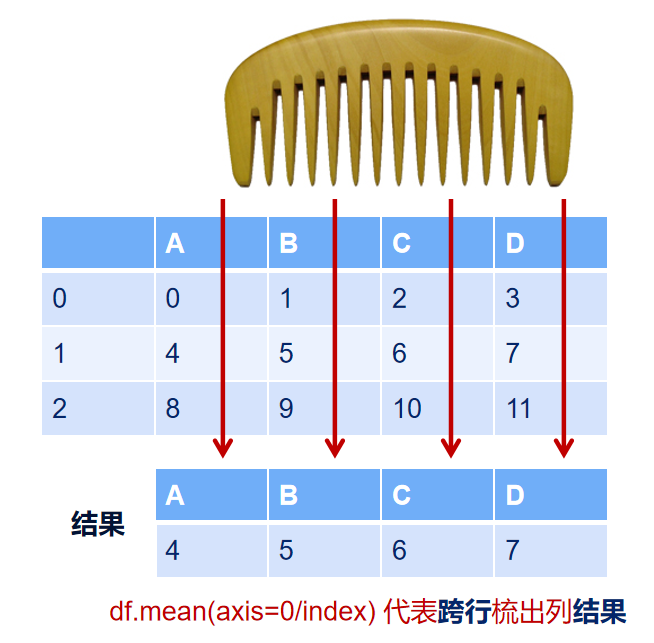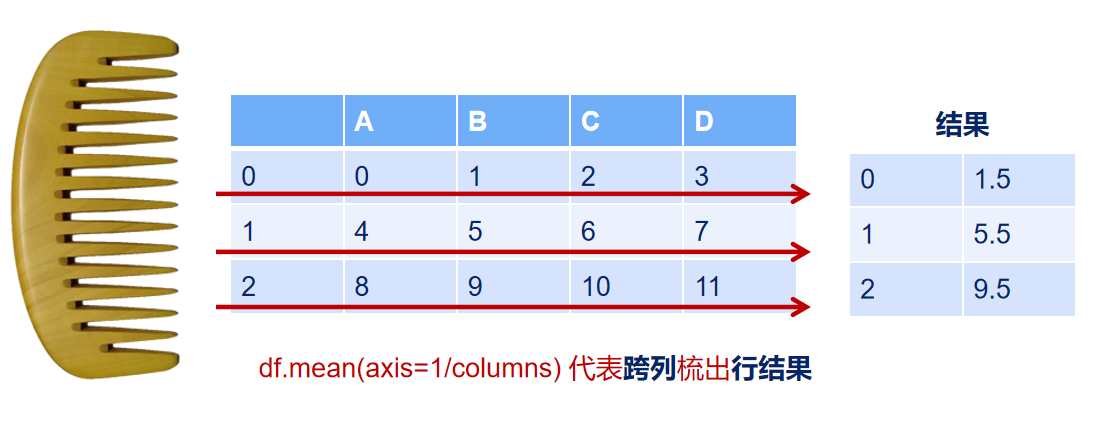# Pandas的axis参数怎么理解？

axis参数非常的让人困惑难以理解，本视频我会用形象化的方式讲解一下这个参数，核心要诀就是axis那个轴会消失！

• axis=0或者”index”：
• 如果是单行操作，就指的是某一行
• 如果是聚合操作，指的是跨行cross rows
• axis=1或者”columns”：
• 如果是单列操作，就指的是某一列
• 如果是聚合操作，指的是跨列cross columns

``````import pandas as pd
import numpy as np
``````
``````df = pd.DataFrame(
np.arange(12).reshape(3,4),
columns=['A', 'B', 'C', 'D']
)
``````
``````df
``````
ABCD
00123
14567
2891011

### 1、单列drop，就是删除某一列

``````# 代表的就是删除某列
df.drop("A", axis=1)
``````
BCD
0123
1567
291011

### 2、单行drop，就是删除某一行

``````df
``````
ABCD
00123
14567
2891011
``````# 代表的就是删除某行
df.drop(1, axis=0)
``````
ABCD
00123
2891011

### 3、按axis=0/index执行mean聚合操作

``````df
``````
ABCD
00123
14567
2891011
``````# axis=0 or axis=index
df.mean(axis=0)
``````
``````A    4.0
B    5.0
C    6.0
D    7.0
dtype: float64
``````### 4、按axis=1/columns执行mean聚合操作

``````df
``````
ABCD
00123
14567
2891011
``````# axis=1 or axis=columns
df.mean(axis=1)
``````
``````0    1.5
1    5.5
2    9.5
dtype: float64
``````### 5、再次举例，加深理解

``````def get_sum_value(x):
return x["A"] + x["B"] + x["C"] + x["D"]

df["sum_value"] = df.apply(get_sum_value, axis=1)
``````
``````df
``````
ABCDsum_value
001236
1456722
289101138